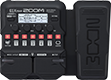# MESA RECTIFIER

Discussion in 'Zoom G1/G1X Four' started by camilomateuso, Mar 6, 2023.

1. MESA RECTIFIERDevice: Zoom G1X Four
Firmware: 2.00

Name on device: RECTIFIER
Optimized for: Phones/Speaker

Effects chain:MESA RECTIFIER

Effect: "ZNR" (Dynamics), active - "yes"
"Detect" = GTRIN
"Depth" = 85
"Threshold" = 20
"Decay" = 0

Effect: "TS Drive" (Overdrive / Distortion), active - "yes"
"Gain" = 25
"Boost" = Off
"Tone" = 25
"Volume" = 82

Effect: "Recti ORG" (Amp simulator), active - "yes"
"Mode" = MDRN
"Bass" = 52
"Middle" = 65
"Treble" = 67
"Presence" = 62
"Gain" = 70
"Volume" = 62

Effect: "RCT4x12" (Cabinet), active - "yes"
"MIC" = ON
"D57: D421" = 0
"Hi" = 50
"Lo" = 50

Effect: "Gt GEQ 7" (Filter), active - "no"
"100" = 0.0
"200" = 0.0
"400" = 0.0
"800" = 0.0
"1.6k" = 0.0
"3.2k" = 0.0
"6.4k" = 0.0
"VOL" = 80

Patch Volume: 85